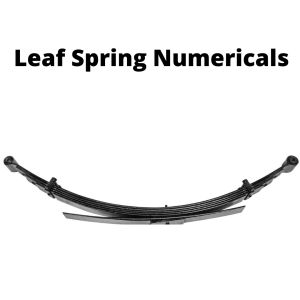Wednesday, October 4, 2023

# Leaf spring Numericals | Important Formulas & Numericals

### 1. Introduction

Springs are elastic members which distort under load and retain their original shape when they are removed. They are used in railway carriers, motor cars, scooters, motorcycles, rickshaws, etc.

### 2. Leaf Spring

Leaf springs are mainly known as laminated or carriage springs.

These types of springs are commonly used in vehicles like trucks, trains, trolleys.They consist of the number of leaves of spring steel held together at the center with clamps.

The plates are provided with a curvature initially and the ends of the top plate are pin-jointed to the chassis of the vehicles.

### 2. Important Formulas

a. Bending Stress

 σ = ( 3WL ) / ( 2Nbt2 )

b. Central Deflection

 δ = ( 3WL3 ) / 8EN bt3 )

c. Moment per plate

 M = (WL) / (4N)

### Numericals

1. A carriage spring is to be 600 mm long and made of 9.5 mm thick steel plates and 50 mm broad. How many plates are required to carry a load of 4.5 kN, without the stress exceeding 230 MN/m2.

What would be central deflection and the initial radius of curvature, if plates straighten under the load? E=200 GN/m2 .

Given,

Span length (L) = 600 mm = 0.6 m

thickness of each plate, (t) = 9.5 = 0.0095 m

Width of each plate, (b) = 50 mm = 0.05m

E = 200 GN/m2

σ = 230 MN/m2

Number of plates, N:

we know,

M = WL / 4N

M = σ x Z

WL / 4N = σ x bt2 / 6

N = (4.5 x 103 x 0.6 x 6) / 4 x 230 x 106 x 0.05 x (0.0095)2

N= 4

σ/y = E/R

we get,

or, 230×106 / (t/2) = 200x 109 / Rc

Rc = 4.13

Central Deflection,

δ = 3WL3 / 8EN bt3

we have,

δ = (3×4.5×103 x 0.63 ) / (8x 200 x 109  x 4 x 0.05 x (0.0095)3

δ = 10.6 mm

2. A Laminated steel spring 1m long is to support the central load of 5.8 KN. If the maximum deflection of spring is not to exceed 45 mm and maximum stress should not exceed 300 MN/m2. Calculate,

1. The thickness of the leaves:

2. Their Number if each plate is to be 80 mm wide.

Given,

E= 200 GN/m2

Span Length ( L ) = 1m

Width of each plate, b= 80 mm =0.08m

δ = 45 mm

σ = 300MN/m2

1. Thickness, t:

Number of Plates:

σ = 3WL / 2Nbt2

or, N t2 = (3 x 5.8 x 103 x 1) /  300 x 106  x 2 x 0.08           ………………. (i)

Also,

δ = 3WL3 / 8EN bt3

Nt3 = (3 x 5.8 x 103 x 13 ) / (0.045x8x 200 x 109 x 0.08)

Nt3 = 3.02 x10-6           ………………. (i)

Dividing eqn ii by i we get,

t = 3.02 x 10-6 / 3.625 x 10-4

t = 8.33 mm

N= 3.02 / t3

N= 5

3. A leaf spring of semi-ellipse type has 11 plates each 9 cm wide and 1.5 cm thick. The length of the spring is 1.5 m. The plates are made of steel having proof stress of 650 MN/m2. To what radius should the plates be bent initially?

From what height can a load of 600 N fall onto the center of the spring, if the maximum stress is to be one-half of the proof stress?

Given,

E = 200 GN/m2

The bending equation is given by,

M/ I = σ/y = E/R

R = E x y / σ

R = E x t/2 / σ

R = 200 x 109 x (0.75/100) / 650 x 106

R= 2.31 m

The stress in the second case is half the proof stress,

650/2 = 325 GN/m2

Then,

σ = 3WL / 2Nbt2

W= ( 2 x 11 x (9/100) x (1.5/100)2 x 325 x10) / 3x 1.5

W = 32.175 KN

δ = 3WL3 / 8EN bt3

δ = ( 3×32175 x1.53 ) / ( 8 x 200 x 109 x 11 x (9/100) x (1.5/100)3 )

δ = 61 mm

P= Falling weight

Let,

Then, work done by the failing weight = P( h + δ )

Wδ / 2 = P( h + δ )

600 (h + 0.061) = (32175 x 0.061) / 2

h = 1.574 m.

 Read More: Difference Between Helical and Leaf springsHe is a founder and lead author of Dream Civil International and his civil engineering research articles has been taken as source by world's top news and educational sites like USA Today, Time, The richest, Wikipedia, etc.
Latest Articles

Related Articles